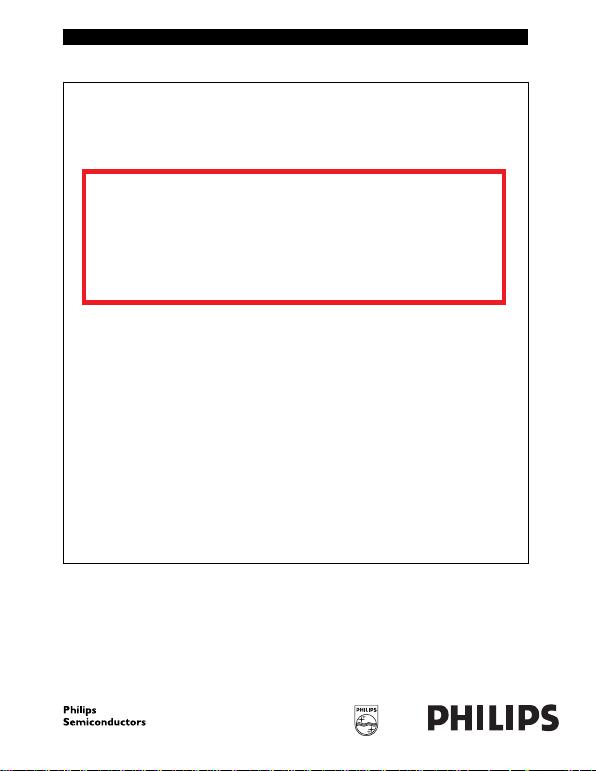DATA SH EET
Product speciﬁcation
File under Integrated Circuits, IC06 December 1990
INTEGRATED CIRCUITS
74HC/HCT30
8-input NAND gate
The IC06 74HC/HCT/HCU/HCMOS Logic Family Specifications
The IC06 74HC/HCT/HCU/HCMOS Logic Package Information
The IC06 74HC/HCT/HCU/HCMOS Logic Package Outlines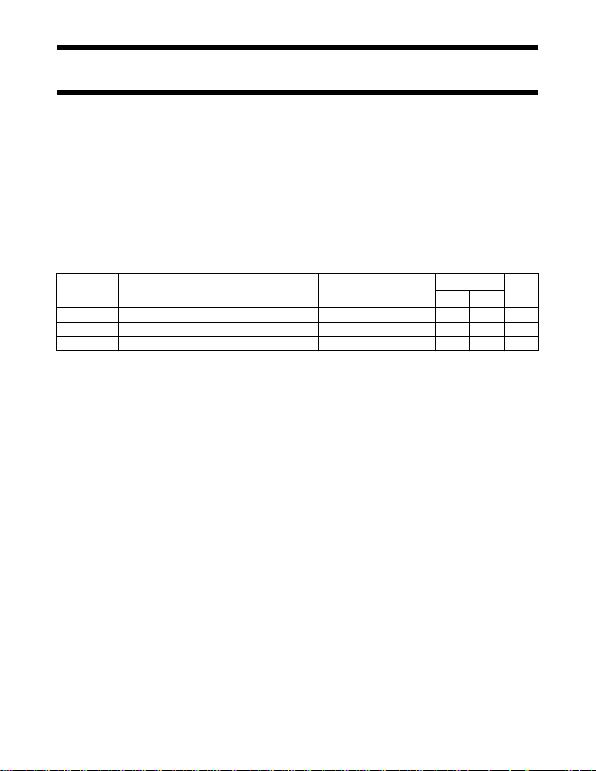December 1990 2
Philips Semiconductors Product speciﬁcation
8-input NAND gate 74HC/HCT30
FEATURES
Output capability: standard
ICC category: SSI
GENERAL DESCRIPTION
The 74HC/HCT30 are high-speed Si-gate CMOS devices and are pin compatible with low power Schottky TTL (LSTTL).
They are specified in compliance with JEDEC standard no. 7A.
The 74HC/HCT30 provide the 8-input NAND function.
QUICK REFERENEC DATA
GND = 0 V; Tamb =25°C; tr=t
f=6ns
Notes
1. CPD is used to determine the dynamic power dissipation (PD in µW):
PD=C
PD ×VCC2×fi+∑(CL×VCC2×fo) where:
fi= input frequency in MHz
fo= output frequency in MHz
(CL×VCC2×fo) = sum of outputs
CL= output load capacitance in pF
VCC = supply voltage in V
2. For HC the condition is VI= GND to VCC
For HCT the condition is VI= GND to VCC 1.5 V
ORDERING INFORMATION
See
“74HC/HCT/HCU/HCMOS Logic Package Information”
.
SYMBOL PARAMETER CONDITIONS TYPICAL UNIT
HC HCT
tPHL/ tPLH propagation delay A, B, C, D, E, F, G, H to Y CL= 15 pF; VCC = 5 V 12 12 ns
CIinput capacitance 3.5 3.5 pF
CPD power dissipation capacitance per gate notes 1 and 2 15 15 pF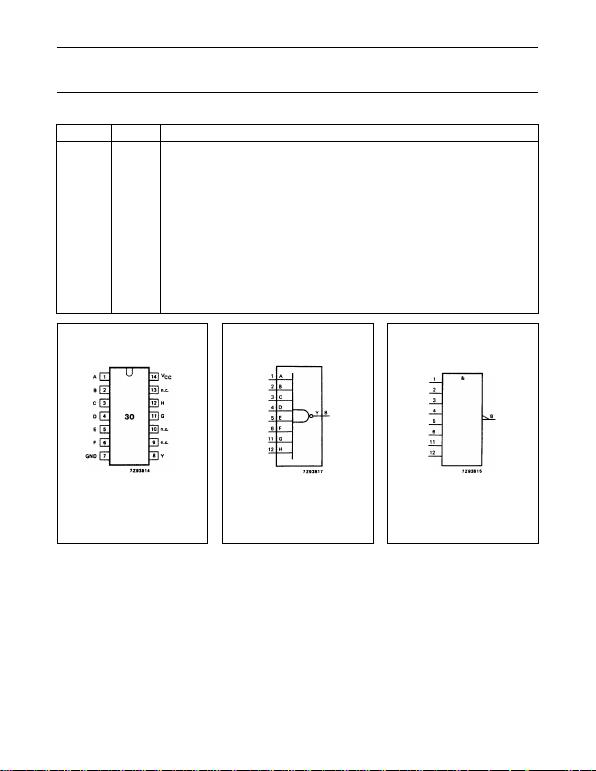December 1990 3
Philips Semiconductors Product speciﬁcation
8-input NAND gate 74HC/HCT30
PIN DESCRIPTION
PIN NO. SYMBOL NAME AND FUNCTION
1 A data input
2 B data input
3 C data input
4 D data input
5 E data input
6 F data input
7 GND ground (0 V)
8 Y data output
9, 10, 13 n.c. not connected
11 G data input
12 H data input
14 VCC positive supply voltage
Fig.1 Pin configuration. Fig.2 Logic symbol. Fig.3 IEC logic symbol.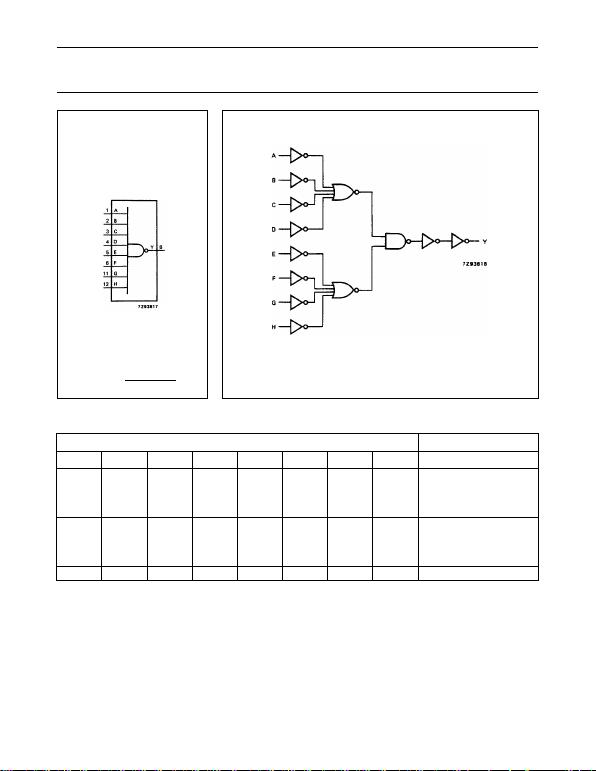December 1990 4
Philips Semiconductors Product speciﬁcation
8-input NAND gate 74HC/HCT30
Fig.4 Functional diagram;
Y=ABCDEFGH. Fig.5 Logic diagram.
FUNCTION TABLE
Notes
1. H = HIGH voltage level
L = LOW voltage level
X = don’t care
INPUTS OUTPUT
ABCDEFGH Y
L
X
X
X
X
L
X
X
X
X
L
X
X
X
X
L
X
X
X
X
X
X
X
X
X
X
X
X
X
X
X
X
H
H
H
H
X
X
X
X
X
X
X
X
X
X
X
X
X
X
X
X
L
X
X
X
X
L
X
X
X
X
L
X
X
X
X
L
H
H
H
H
HHHHHHHH L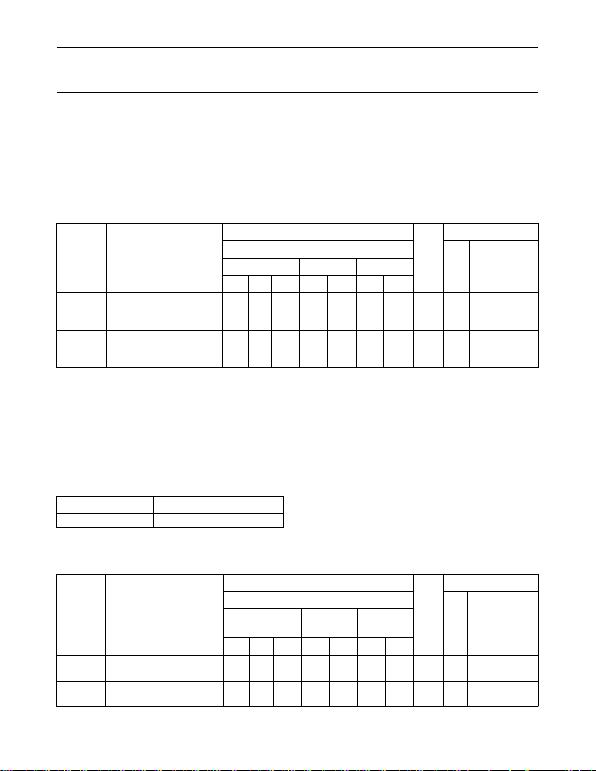December 1990 5
Philips Semiconductors Product speciﬁcation
8-input NAND gate 74HC/HCT30
DC CHARACTERISTICS FOR 74 HC
For the DC characteristics see
“74HC/HCT/HCU/HCMOS Logic Family Specifications”
.
Output capability: standard
ICC category: SSI
AC CHARACTERISTICS FOR 74HC
GND = 0 V; tr=t
f= 6 ns; CL=50pF
DC CHARACTERISTICS FOR 74HCT
For the DC characteristics see
“74HC/HCT/HCU/HCMOS Logic Family Specifications”
.
Output capability: standard
ICC category: SSI
Note to HCT types
The value of additional quiescent supply current (ICC) for a unit load of 1 is given in the family specifications.
To determine ICC per input, multiply this value by the unit load coefficient shown in the table below.
AC CHARACTERISTICS FOR 74HCT
GND = 0 V; tr=t
f= 6 ns; CL=50pF
SYMBOL PARAMETER
Tamb (°C)
UNIT
TEST CONDITIONS
74HC VCC
(V) WAVEFORMS+25 40 to +85 40 to +125
min. typ. max. min. max. min. max.
tPHL/ tPLH propagation delay
A, B, C, D, E, F, G, H to Y 41
15
12
130
26
22
165
33
28
195
39
33
ns 2.0
4.5
6.0
Fig.6
tTHL/ tTLH output transition time 19
7
6
75
15
13
95
19
16
110
22
19
ns 2.0
4.5
6.0
Fig.6
A, B, C, D, E, F, G, H 0.60
SYMBOL PARAMETER
Tamb (°C)
UNIT
TEST CONDITIONS
74HCT
VCC
(V) WAVEFORMS+25 40 to +85 40
to +125
min. typ. max. min. max. min. max.
tPHL/ tPLH propagation delay
A, B, C, D, E, F, G, H to Y 16 28 35 42 ns 4.5 Fig.6
tTHL/ tTLH output transition time 7 15 19 22 ns 4.5 Fig.6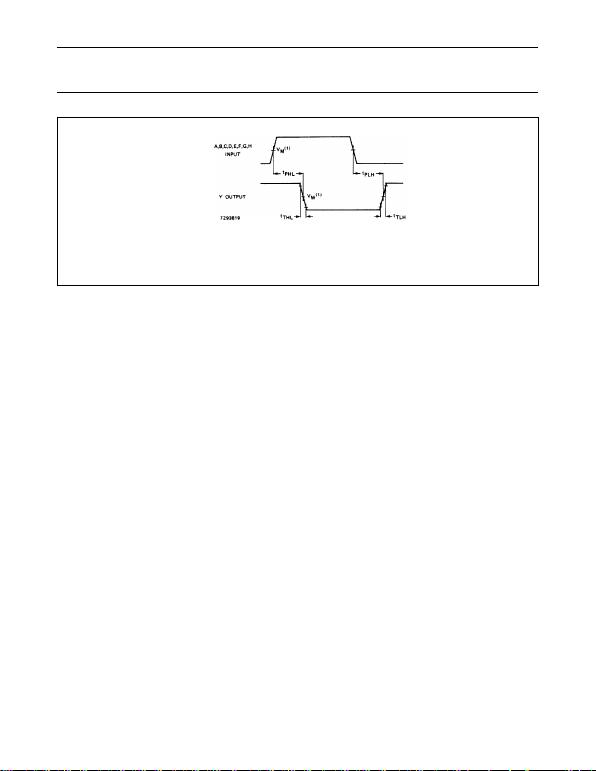December 1990 6
Philips Semiconductors Product speciﬁcation
8-input NAND gate 74HC/HCT30
AC WAVEFORMS
PACKAGE OUTLINES
See
“74HC/HCT/HCU/HCMOS Logic Package Outlines”
.
Fig.6 Waveforms showing the input (A, B, C, D, E, F, G, H) to output (Y) propagation delays and the output
transition times.
(1) HC : VM= 50%; VI= GND to VCC.
HCT: VM= 1.3 V; VI= GND to 3 V.